# Understand the principle of affine transformation and python implementation code

2022-02-02 04:21:49

Affine transformation of images is often used in data enhancement of computer vision , By affine transformation, we mean rigid body transformation , That is, two parallel lines are still parallel after affine transformation , Perspective transformation is not rigid body transformation , Beyond the scope of this article . Usually flip 、 translation 、 rotate 、 Shrinkage 、 Any combination of staggered transformation belongs to affine transformation . Affine transformation can be expressed by the following formula ：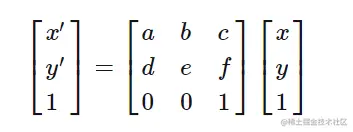Where the rotation angle acts on a b d e,scale Act on a e, Shear transformation acts on b d, Translation acts on c f, As shown in the figure below ：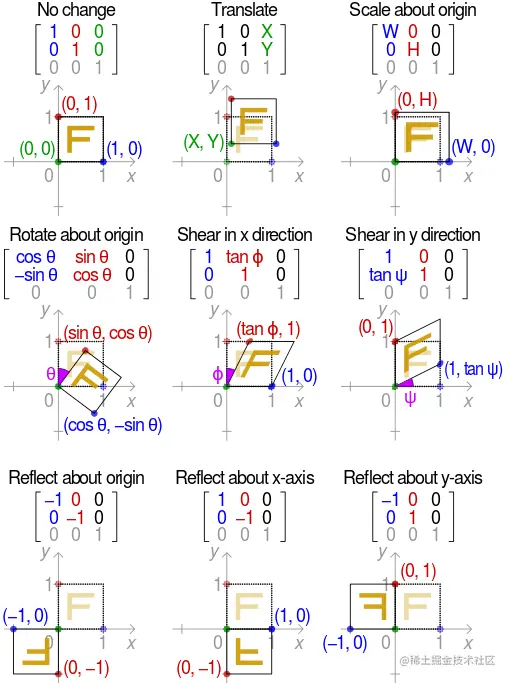## 1. cv2.getRotationMatrix2D Detailed explanation

opencv Of cv2.getRotationMatrix2D Function can obtain the rotation transformation matrix . Enter the coordinates of the center point (centerX,centerY), Rotation Angle θ And scaling 1, give M Transformation matrix ：

$\left[ \begin{matrix} cosθ & -sinθ & (1 - cosθ) * centerX + sinθ * centerY \\ sinθ & cosθ & (1 - cosθ) * centerY + sinθ * centerX \\ 0 & 0 & 1 \end{matrix} \right]$

How is this affine transformation matrix derived ？ Affine transformation matrix adopts polar coordinate system, which is easy to reason . Let's first rotate a point based on the origin , Here's the picture , take V1 The dot rotates counterclockwise θ Angle to V2 spot , Let's first assume that the scale is 1.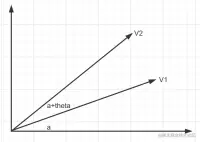V1 The angle between the point and the origin line and the horizontal line a,V2 The angle between the point and the origin line and the horizontal line b=a+θ. Calculate the rotation transformation matrix ： remember V1=(x1, y1), V2=(x2, y2)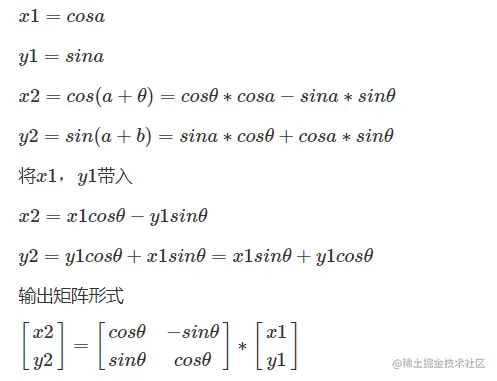But usually we rotate based on the center point , If you need to go around any point （tx,ty） rotate , We can
1. First translate the rotation point to the origin
2. Then perform the above rotation operation
3. Press 1 The reverse operation of translation goes back
You can get the transformation matrix of rotating points around any point ：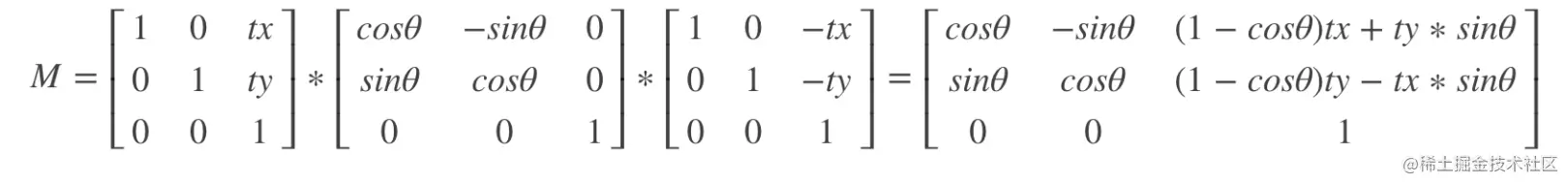## 2. warpAffine operation

### 2.1 obtain M Affine transformation of matrix

Get the transformation matrix M, Each point of the image is M The rotated image can be obtained by transformation , This step can be done by opencv Of warpAffine obtain . But through the above operation , After rotation, the large image will lose information , As shown in the figure below ：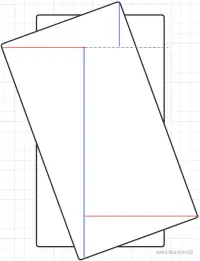### 2.2 Expand the canvas

With the same canvas size , There will be some images beyond , Display incomplete , So we need to expand the canvas to ： The new height is composed of two blue lines in the picture :new_H=int(w∗abs(sin(radias(angle)))+h∗abs(cos(radias(angle))))

The new width consists of two red lines in the picture : new_W=int(h∗fabs(sin(radians(angle)))+w∗fabs(cos(radians(angle))))

The new canvas expansion is based on the expansion of the upper left corner of the original image . therefore , In the transformation matrix M On , We can adjust the translation parameters ：

M[0,2]+=(new_W−w)/2

M[1,2]+=(new_H−h)/2

## 3. Image affine transformation code

Talk is cheap, show me the code. Go straight to the code ：

def affine_transform(image, angle, scale=1.0):
h, w = image.shape[:2]
center_x, center_y = width // 2, height // 2

M = cv2.getRotationMatrix2D((center_x, center_y), angle, scale)
cos = np.abs(M[0, 0])
sin = np.abs(M[0, 1])

new_h = int(h * cos + w * sin)
new_w = int(h * sin + w * cos)
M[0, 2] += (new_w − w) / 2
M[0, 1] += (new_h − h) / 2

new_image = cv2.warpAffine(image, M, (new_w, new_h))
return new_image
Copy code 

## 4. Single point affine transformation code

When we perform affine transformation data enhancement , The coordinate points of the target need to be transformed accordingly .

def affine_points(points, center_x, center_y, h, w, scale=1.0):
points = np.array(points, dtype=np.float32)
points = np.hstack((points, np.ones((points.shape, 1))))

M = cv2.getRotationMatrix2D((center_x, center_y), angle, scale)
cos = np.abs(M[0, 0])
sin = np.abs(M[0, 1])

new_h = int(h * cos + w * sin)
new_w = int(h * sin + w * cos)
M[0, 2] += (new_w − w) / 2
M[0, 1] += (new_h − h) / 2

new_points = np.dot(M, points.T).T
return new_points
Copy code